# Examples for using `geomnet`

#### 2020-11-25

``````library(ggplot2)
library(geomnet)
data(blood)
p <- ggplot(data = blood\$edges, aes(from_id = from, to_id = to))
p + geom_net(vertices=blood\$vertices, aes(colour=..type..)) + theme_net()``````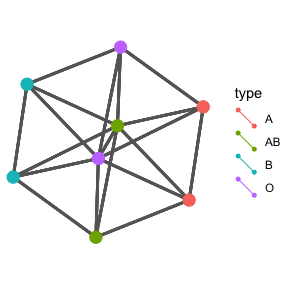``````#'
bloodnet <- fortify(as.edgedf(blood\$edges), blood\$vertices)``````
``````## Using from as the from node column and to as the to node column.
## If this is not correct, rewrite dat so that the first 2 columns are from and to node, respectively.``````
``## Joining edge and node information by from_id and label respectively.``
``````p <- ggplot(data = bloodnet, aes(from_id = from_id, to_id = to_id))
p + geom_net()``````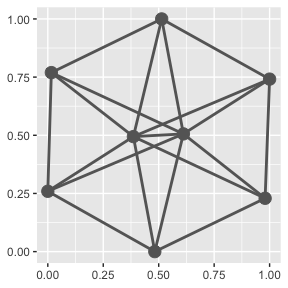``p + geom_net(aes(colour=rho)) + theme_net()``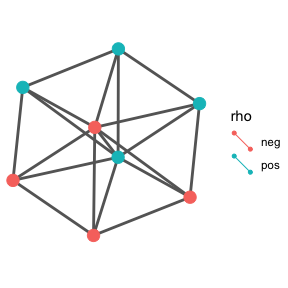``p + geom_net(aes(colour=rho), labelon=TRUE, vjust = -0.5)``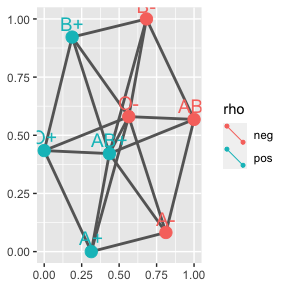``````p + geom_net(aes(colour=rho, linetype = group_to, label = from_id),
vjust=-0.5, labelcolour="black", directed=TRUE) +
theme_net()``````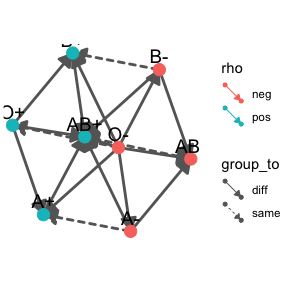``p + geom_net(colour = "orange", layout.alg = 'circle', size = 6)``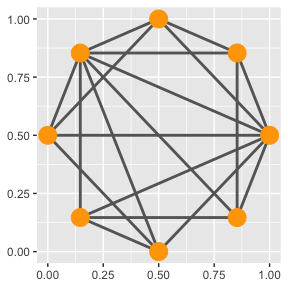``p + geom_net(colour = "orange", layout.alg = 'circle', size = 6, linewidth=.75)``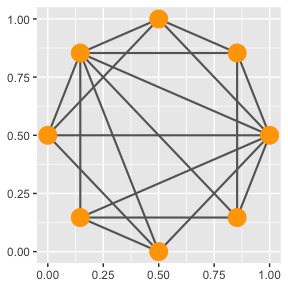``````p + geom_net(colour = "orange", layout.alg = 'circle', size = 0, linewidth=.75,
directed = TRUE)``````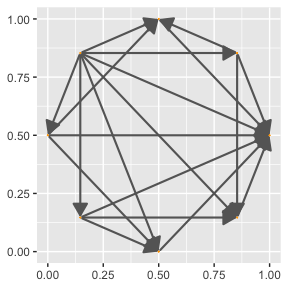``````p + geom_net(aes(size=Predominance, colour=rho, shape=rho, linetype=group_to),
linewidth=0.75, labelon =TRUE, labelcolour="black") +
facet_wrap(~Ethnicity) +
scale_colour_brewer(palette="Set2")``````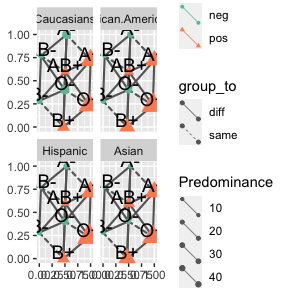``````gg <- ggplot(data = blood\$edges, aes(from_id = from, to_id = to)) +
geom_net(colour = "darkred", layout.alg = "circle", labelon=TRUE, size = 15,
directed = TRUE, vjust = 0.5, labelcolour = "grey80",
arrowsize = 1.5, linewidth = 0.5, arrowgap = 0.05,
selfloops = TRUE, ecolour = "grey40") +
theme_net()
gg``````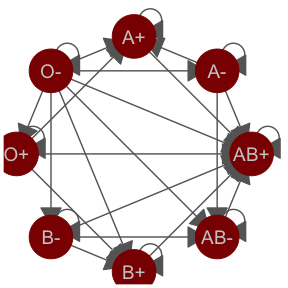``````dframe <- ggplot_build(gg)\$data[] # contains calculated node and edge values
#'
#Madmen Relationships
data(madmen)
MMnet <- fortify(as.edgedf(madmen\$edges), madmen\$vertices)``````
``````## Using Name1 as the from node column and Name2 as the to node column.
## If this is not correct, rewrite dat so that the first 2 columns are from and to node, respectively.
## Joining edge and node information by from_id and label respectively.``````
``````p <- ggplot(data = MMnet, aes(from_id = from_id, to_id = to_id))
p + geom_net(labelon=TRUE)``````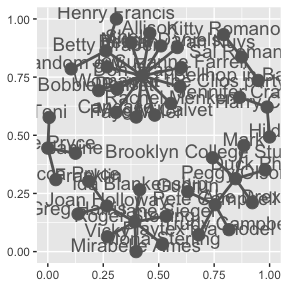``p + geom_net(aes(colour=Gender), size=6, linewidth=1, labelon=TRUE, fontsize=3, labelcolour="black")``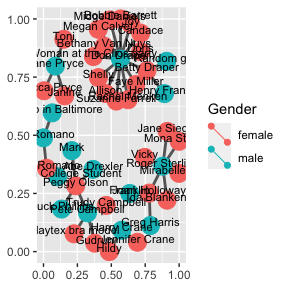``````p + geom_net(aes(colour=Gender), size=6, linewidth=1, labelon=TRUE, labelcolour="black") +
scale_colour_manual(values=c("#FF69B4", "#0099ff")) + xlim(c(-.05,1.05))``````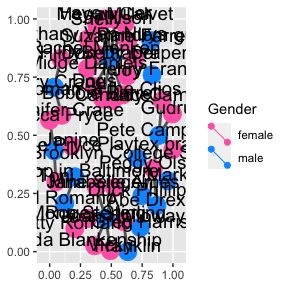``````p + geom_net(aes(colour=Gender), size=6, linewidth=1, directed=TRUE, labelon=TRUE,
arrowgap=0.01, labelcolour="black") +
scale_colour_manual(values=c("#FF69B4", "#0099ff")) + xlim(c(-.05,1.05))``````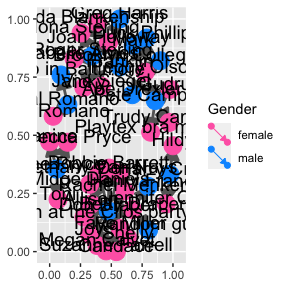``````#'
p <- ggplot(data = MMnet, aes(from_id = from_id, to_id = to_id))
# alternative labelling: specify label aesthetic.
p + geom_net(aes(colour=Gender, label=Gender), size=6, linewidth=1, fontsize=3,
labelcolour="black")``````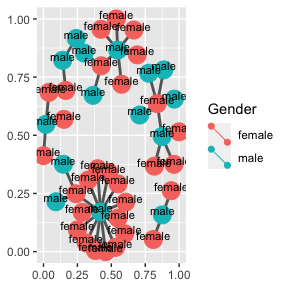``````#'
## visualizing ggplot2 theme elements
data(theme_elements)
TEnet <- fortify(as.edgedf(theme_elements\$edges[,c(2,1)]), theme_elements\$vertices)``````
``````## Using parent as the from node column and child as the to node column.
## If this is not correct, rewrite dat so that the first 2 columns are from and to node, respectively.``````
``## Joining edge and node information by from_id and name respectively.``
``````ggplot(data = TEnet, aes(from_id = from_id, to_id = to_id)) +
geom_net(labelon=TRUE, vjust=-0.5)``````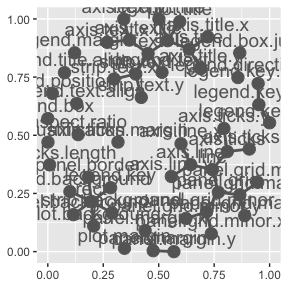``````#'
## emails example from VastChallenge 2014
# care has to be taken to make sure that for each panel all nodes are included with
# the necessary information.
# Otherwise line segments show on the plot without nodes.
emailedges <- as.edgedf(subset(email\$edges, nrecipients < 54))``````
``````## Using From as the from node column and eID as the to node column.
## If this is not correct, rewrite dat so that the first 2 columns are from and to node, respectively.``````
``emailnet <- fortify(emailedges, email\$nodes)``
``## Joining edge and node information by from_id and label respectively.``
``````#no facets
ggplot(data = emailnet, aes(from_id = from_id, to_id = to_id)) +
geom_net(aes(colour= CurrentEmploymentType), linewidth=0.5) +
scale_colour_brewer(palette="Set2")``````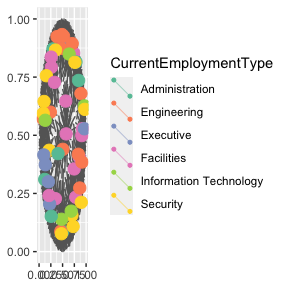``````#facet by day
#'
emailnet <- fortify(emailedges, email\$nodes, group = "day")``````
``## Joining edge and node information by from_id and label respectively.``
``````ggplot(data = emailnet, aes(from_id = from, to_id = to_id)) +
geom_net(aes(colour= CurrentEmploymentType), linewidth=0.5, fiteach=TRUE) +
scale_colour_brewer(palette="Set2") +
facet_wrap(~day, nrow=2) + theme(legend.position="bottom")``````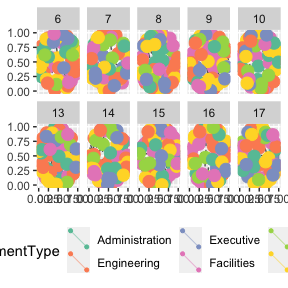``````ggplot(data = emailnet, aes(from_id = from, to_id = to_id)) +
geom_net(aes(colour= Citize``````